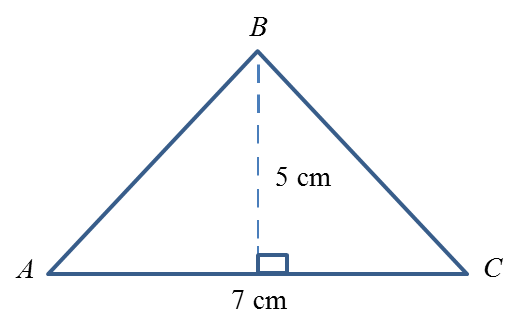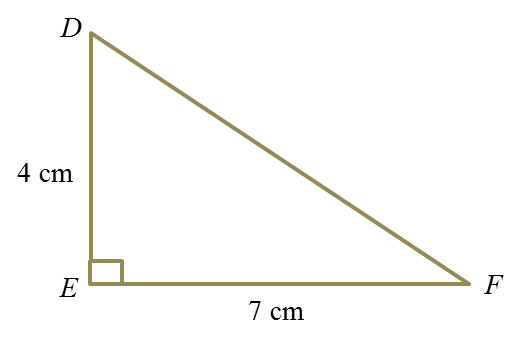# 3.6 Deduction and Induction

3.6 Deduction and Induction

(A) Reasoning by Deduction and Induction
1. Reasoning by deduction is a process of making a conclusion for a specific case based on a given general statement.

2.
Reasoning by induction is a process of making a generalization based on specific cases.

 Maths Tip 1. General statement  →  Special conclusion  → Deduction 2. Specific cases  →  General conclusion  →  Induction
Example:
Determine whether the following conclusion is made based on a deductive reasoning or inductive reasoning.

(a)
Area of triangle = ½ × Base × Height
(i)Area of ∆ ABC
= ½ × 7cm × 5cm
= 17.5 cm2

(ii)Area of ∆ DEF
= ½ × 7cm × 4cm
= 14 cm2

(b)
1 = 7 (1)2 – 6
22 = 7 (2)2 – 6
57 = 7 (3)2 – 6
106 = 7 (4)2 – 6
7n2 – 6, n = 1, 2, 3, 4…

Solution:
(a)
The specific conclusion is made based on a general statement ~ Area of triangle = ½ × Base × Height. Therefore, the conclusion is made based on deductive reasoning.

(b)

The general conclusion 7n2 – 6, n = 1, 2, 3, 4… is made based on specific cases. Therefore, the conclusion is based on inductive reasoning.